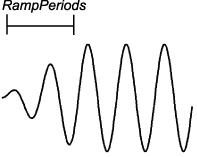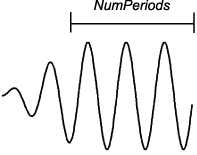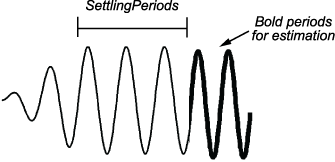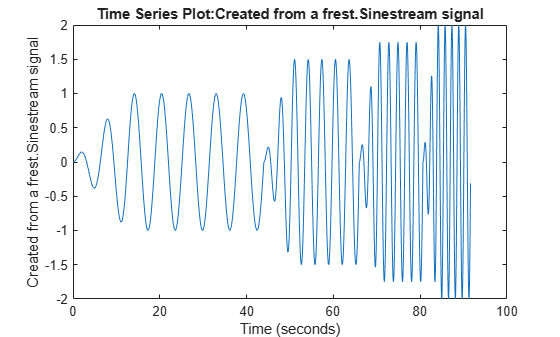Documentation

# frest.Sinestream

Package: frest

Signal containing series of sine waves

## Syntax

```input = frest.Sinestream(sys) input = frest.Sinestream('OptionName',OptionValue) ```

## Description

`input = frest.Sinestream(sys)` creates a signal with a series of sinusoids based on the dynamics of a linear system `sys`.

`input = frest.Sinestream('OptionName',OptionValue)` creates a signal with a series of sinusoids, where each sinusoid frequency lasts for a specified number of periods, using the options specified by comma-separated name/value pairs.

To view a plot of your input signal, type `plot(input)`. To obtain a timeseries for your input signal, use the `generateTimeseries` command.

## Input Arguments

`sys`

Linear system for creating a sinestream signal based on the dynamic characteristics of this system. You can specify the linear system based on known dynamics using `tf`, `zpk`, or `ss`. You can also obtain the linear system by linearizing a nonlinear system.

The resulting sinestream signal automatically sets these options based on the linear system:

• `'Frequency'` are the frequencies at which the linear system has interesting dynamics.

• `'SettlingPeriods'` is the number of periods it takes the system to reach steady state at each frequency in `'Frequency'`.

• `'NumPeriods'` is (3 + `SettlingPeriods`) to ensure that each frequency excites the system at specified amplitude for at least three periods.

• For discrete systems only, `'SamplesPerPeriod'` is set such that all frequencies have the same sample time as the linear system.

Other sinestream options have default values.

`'OptionName',OptionValue`

Signal characteristics, specified as comma-separated option name and option value pairs.

Option NameOption Value
`'Frequency'`

Signal frequencies, specified as either a scalar or a vector of frequency values.

Default: `logspace(1,3,50)`

`'Amplitude'`

Signal amplitude at each frequency, specified as either:

• Scalar to set all frequencies to same value

• Vector to set each frequencies to a different value

Default: `1e-5`

`'SamplesPerPeriod'`

Number of samples for each period for each signal frequency, specified as either:

• Scalar to set all frequencies to same value

• Vector to set each frequencies to a different value

Default: `40`

`'FreqUnits'`

Frequency units:

• `'rad/s'` — Radians per second

• `'Hz'` — Hertz

Default: `'rad/s'`

`'RampPeriods'`

Number of periods for ramping up the amplitude of each sine wave to its maximum value, specified as either:

• Scalar to set all frequencies to same value

• Vector to set each frequencies to a different value

Use this option to ensure a smooth response when your input amplitude changes.

Default: `0``'NumPeriods'`

Number of periods each sine wave is at maximum amplitude, specified as either:

• Scalar to set all frequencies to same value

• Vector to set each frequencies to a different value

Default: ```max(3 - RampPeriods + SettlingPeriods, 2)````'SettlingPeriods'`

Number of periods corresponding to the transient portion of the simulated response at a specific frequency, before the system reaches steady state, specified as either:

• Scalar to set all frequencies to same value

• Vector to set each frequencies to a different value

Before performing the estimation, `frestimate` discards this number of periods from the output signals.

Default: `1``'ApplyFilteringInFRESTIMATE'`

Frequency-selective FIR filtering of the input signal before estimating the frequency response using `frestimate`.

• `'on'` (default)

• `'off'`

For more information, see the `frestimate` algorithm.

`'SimulationOrder'`

The order in which `frestimate` injects the individual frequencies of the input signal into your Simulink® model during simulation.

• `'Sequential'` (default) — `frestimate` injects one frequency after the next into your model in a single Simulink simulation using variable sample time. To use this option, your Simulink model must use a variable-step solver.

• `'OneAtATime'``frestimate` injects each frequency during a separate Simulink simulation of your model. Before each simulation, `frestimate` initializes your Simulink model to the operating point specified for estimation. If you have Parallel Computing Toolbox™ installed, you can run each simulation in parallel to speed up estimation using parallel computing. For more information, see Speeding Up Estimation Using Parallel Computing.

## Examples

collapse all

Create a sinestream input signal for estimation by specifying the frequencies for the signal. Also, specify the amplitude, the number of ramp-up periods, the number of settling periods, and the total number of periods after the ramp-up.

To specify the frequencies, use a vector of frequencies.

`freqs = linspace(1,4,4);`

To specify the other parameters, use a scalar to use the same parameter value for every frequency. To use different values for each frequency, use a vector of the same length as `freqs`. For this example, use increasing amplitudes at each frequency, but keep the number of ramp-up periods, number of settling periods, and the number of periods after ramp-up constant.

```amps = [1 1.5 1.75 2]; ramp = 2; settle = 3; pds = 5; input = frest.Sinestream('Frequency',freqs,... 'Amplitude',amps,... 'RampPeriods',ramp,... 'SettlingPeriods',settle,... 'NumPeriods',pds);```

Examine the resulting sinestream signal.

`plot(input)`When your sinestream signal covers a wide range of frequencies, it can be inefficient to use the same sample time across all frequencies. For that reason, `frest.Sinestream` by default uses a fixed number of samples at each frequency. You can specify that number with a scalar value, or use a vector to provide a different number of samples at each frequency. (To create a sinestream signal with a fixed sample time across the entire signal, use `frest.createFixedTsSinestream`. This option is useful when the input linearization point for estimation is on a discrete-time signal.)

Create a sinusoidal input signal with the following characteristics:

• 50 frequencies spaced logarithmically between 10 Hz and 1000 Hz

• Amplitude of 1e-3 at all frequencies

• Sampled with a frequency 10 times the frequency of the signal (meaning ten samples per period)

```input = frest.Sinestream('Amplitude',1e-3,... 'Frequency',logspace(1,3,50),... 'SamplesPerPeriod',10,... 'FreqUnits','Hz');```

Create a sinestream input signal based on the dynamics of a linear system. This approach is useful when you are using frequency response estimation to validate the linearization of your model.

Open a Simulink model.

```model = 'watertank'; open_system(model) ```For this example, linearize the model at a steady-state operating point to obtain a state-space model you can use to initialize the sinestream signal.

```io(1)=linio('watertank/PID Controller',1,'input'); io(2)=linio('watertank/Water-Tank System',1,'openoutput'); watertank_spec = operspec(model); opOpts = findopOptions('DisplayReport','off'); op = findop(model,watertank_spec,opOpts); sys = linearize(model,op,io); ```

Create the sinestream signal.

```input = frest.Sinestream(sys); ```

`frest.Sinestream` chooses frequencies based on the system dynamics. It also automatically initializes other parameters of the sinestream signal.

```input ```
``` The sinestream input signal: Frequency : [0.0015811;0.0026375;0.0043996;0.007339 ...] (rad/s) Amplitude : 1e-05 SamplesPerPeriod : 40 NumPeriods : [4;4;4;4 ...] RampPeriods : 0 FreqUnits (rad/s,Hz): rad/s SettlingPeriods : [1;1;1;1 ...] ApplyFilteringInFRESTIMATE (on/off) : on SimulationOrder (Sequential/OneAtATime): Sequential ```

You can change properties of the signal using dot notation. For instance, increase the signal amplitude.

```input.Amplitude = 3e-5 ```
``` The sinestream input signal: Frequency : [0.0015811;0.0026375;0.0043996;0.007339 ...] (rad/s) Amplitude : 3e-05 SamplesPerPeriod : 40 NumPeriods : [4;4;4;4 ...] RampPeriods : 0 FreqUnits (rad/s,Hz): rad/s SettlingPeriods : [1;1;1;1 ...] ApplyFilteringInFRESTIMATE (on/off) : on SimulationOrder (Sequential/OneAtATime): Sequential ```

#### Introduced in R2009b

##### SupportGet trial now